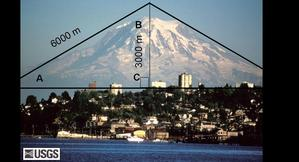# Basic trig functions - practice problems

These problems are designed to help you learn basic trigonometry ("trig") functions and how to use your calculator correctly.
Try solving these on your own (without peaking at the solutions). You may want to download a sheet with all the questions (Acrobat (PDF) 33kB Jul25 09) to print out and try. If you need help, look at the hidden solutions. You may use a calculator.

## Calculating sine, cosine, and tangent

Problem 1. The angle of repose for sand is typically about 35°. What is the sine of this angle?

Problem 2. When driving, a steep hill is typically only 12°. What is the cosine of this angle?

Problem 3. The angle that waves hit a shoreline is 75°. What is the tangent of this angle?

For the next three problems, use the photo of Mount Rainier below.Problem 4.What is the sine of angle A?

Problem 5. Determine angle A in degrees.

Problem 6.
What is the cosine of angle B?